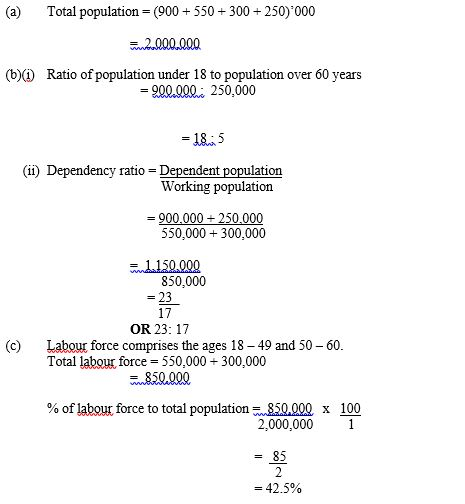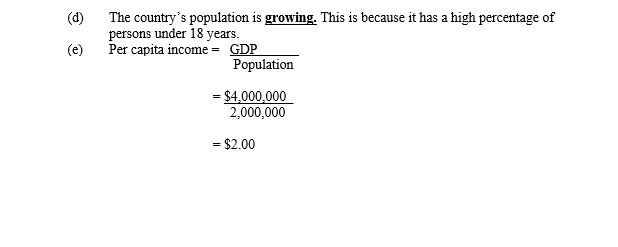Question 1

The following data shows the budget of a hypothetical country in 2006. Use the information in the table to answer the questions that follow:

 Age (years) 0 - 17 18 - 49 50 - 60 60 and above Population (‘000) 900 550 300 250

What is the country’s total population?

(b)         Calculate:
(i)         the ratio of population under 18 to population over 60 years;

(ii)        the dependency ratio.
(c)         What percentage of the population constitutes the labour force?

(d)         Explain whether the population is growing or declining.

(e)         Given that the GDP of the country in 2009 is \$ 4,000,000, what is the per capita income of the country?

This is one of the data response questions and it attracted more than three quarter of the candidates, most of who performed above average. The question required the candidates to calculate the total population, dependency ratio, labour force, per capita income of a hypothetical country and explain whether her population is growing or declining. Most candidates were able to calculate what was required but were unable score maximum marks because most of them disregarded the thousands (‘000) in their computation in the table given.
The candidates were expected to provide the following answers to score higher marks.